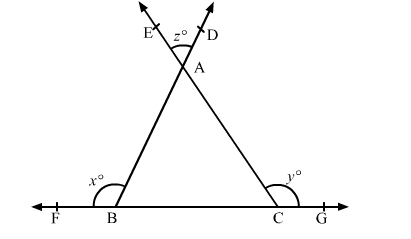# In the given figure, two rays BD and CE intersect at a point A.

Question:

In the given figure, two rays BD and CE intersect at a point A. The side BC of ∆ABC have been produced on both sides to points F and G respectively. If ∠ABF x°, ∠ACG y° and ∠DAE z° then = ?
(a) x + y – 180
(b) x + y + 180
(c) 180 – (x + y)
(d) x + y + 360°Solution:

In the given figure, ∠ABF + ∠ABC = 180°         (Linear pair of angles)

∴ x° + ∠ABC = 180°

⇒ ∠ABC = 180° − x°        .....(1)

Also, ∠ACG + ∠ACB = 180°         (Linear pair of angles)

∴ y° + ∠ACB = 180°

⇒ ∠ACB = 180° − y°        .....(2)

Also, ∠BAC = ∠DAE = z°       .....(3)         (Vertically opposite angles)

In ∆ABC,

∠BAC + ∠ABC + ∠ACB = 180°       (Angle sum property)

∴ z° + 180° − x° + 180° − y° = 180°        [Using (1), (2) and (3)]

⇒ z = x + y − 180

Hence, the correct answer is option (a)